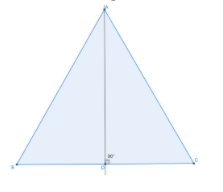Courses
Courses for Kids
Free study material
Free LIVE classes
MoreLIVE
Join Vedantu’s FREE Mastercalss

# In an equilateral triangle ABC, AD$\bot$ BC. Prove that $A{D^2} = 3B{D^2}$.Verified
361.8k+ views
Hint: The altitude is also the median for an equilateral triangle. Use this property and the Pythagoras theorem (the square of the hypotenuse is the sum of the squares of the other two sides, in a right-angled triangle) to prove what is required.

ABC is an equilateral triangle. AD is perpendicular to BC.
AD$\bot$ BC. So, AD is the altitude of triangle ABC.
Let us draw the diagram.We know that, in an equilateral triangle the altitude is also the median.
Hence, D is the midpoint of BC.
BD=DC
We can also say that BD is half the length of BC.
$BD = \dfrac{1}{2}BC$ …(1)
We will use the Pythagoras theorem which states that the square of the hypotenuse is the sum of the squares of the other two sides, in a right-angled triangle.
In triangle ADB, AB is the hypotenuse.
$A{B^2} = B{D^2} + A{D^2}$
By rearranging we get,
$A{D^2} = A{B^2} - B{D^2}$ …(2)
We know that, in an equilateral triangle, all the sides are equal.
So, BC=AB …(3)
Using (3) in (1) we get,
$BD = \dfrac{1}{2}AB$
By cross-multiplying we get,
$AB = 2BD$ …(4)
Using (4) in (1) we get,
$A{D^2} = {(2BD)^2} - B{D^2} \\ A{D^2} = 4B{D^2} - B{D^2} \\ A{D^2} = 3B{D^2} \\$
Hence, $A{D^2} = 3B{D^2}$ is proved.

Note: In problems where we have to prove LHS=RHS, start from the LHS and take the proof in that direction as expressing the terms in terms of what is given in the RHS. This will lead to getting the correct RHS. Reasons must be written for each step while proving.
Last updated date: 19th Sep 2023
Total views: 361.8k
Views today: 3.61k